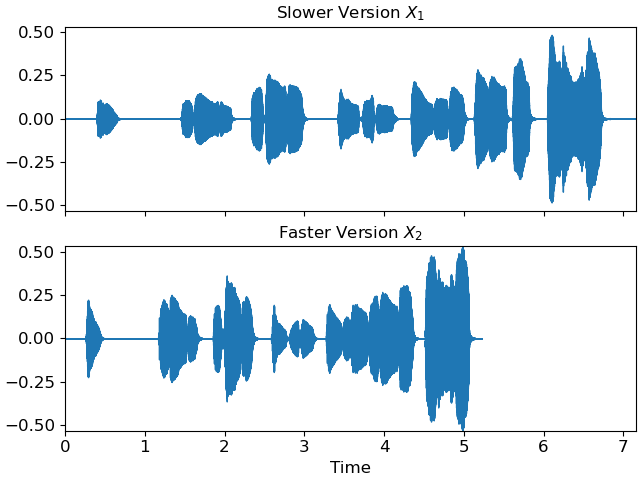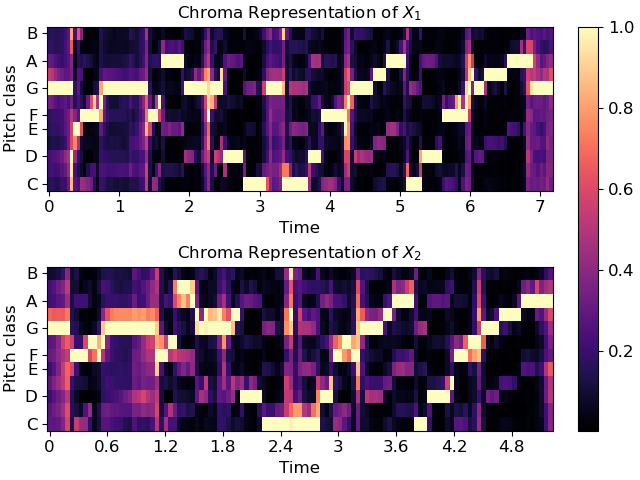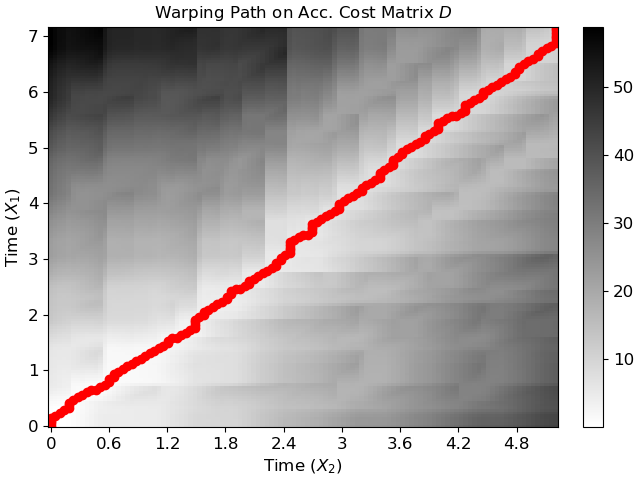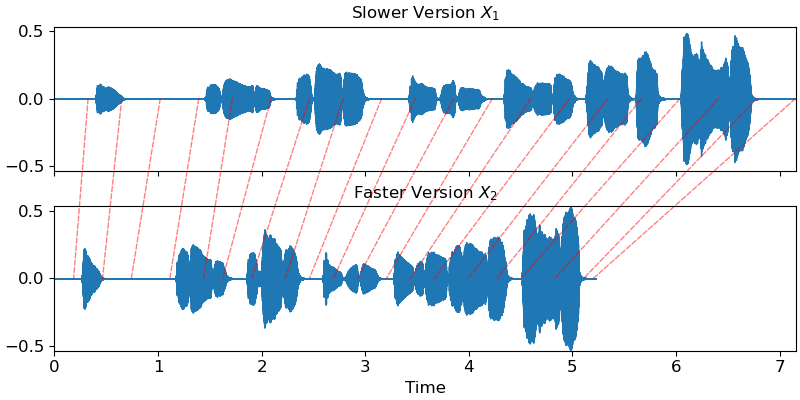Caution

You're reading the documentation for a development version. For the latest released version, please have a look at 0.10.1.

# Music Synchronization with Dynamic Time Warping

In this short tutorial, we demonstrate the use of dynamic time warping (DTW) for music synchronization which is implemented in `librosa`.

We assume that you are familiar with the algorithm and focus on the application. Further information about the algorithm can be found in the literature, e. g. .

Our example consists of two recordings of the first bars of the famous brass section lick in Stevie Wonder’s rendition of “Sir Duke”. Due to differences in tempo, the first recording lasts for ca. 7 seconds and the second recording for ca. 5 seconds. Our objective is now to find an alignment between these two recordings by using DTW.

```# Code source: Stefan Balke
# sphinx_gallery_thumbnail_number = 4

import numpy as np
import matplotlib
import matplotlib.pyplot as plt

import librosa
```

First, let’s load a first version of our audio recordings.

```x_1, fs = librosa.load('audio/sir_duke_slow.ogg')
# And a second version, slightly faster.

fig, ax = plt.subplots(nrows=2, sharex=True, sharey=True)
librosa.display.waveshow(x_1, sr=fs, ax=ax)
ax.set(title='Slower Version \$X_1\$')
ax.label_outer()

librosa.display.waveshow(x_2, sr=fs, ax=ax)
ax.set(title='Faster Version \$X_2\$')
```## Extract Chroma Features

```hop_length = 1024

x_1_chroma = librosa.feature.chroma_cqt(y=x_1, sr=fs,
hop_length=hop_length)
x_2_chroma = librosa.feature.chroma_cqt(y=x_2, sr=fs,
hop_length=hop_length)

fig, ax = plt.subplots(nrows=2, sharey=True)
img = librosa.display.specshow(x_1_chroma, x_axis='time',
y_axis='chroma',
hop_length=hop_length, ax=ax)
ax.set(title='Chroma Representation of \$X_1\$')
librosa.display.specshow(x_2_chroma, x_axis='time',
y_axis='chroma',
hop_length=hop_length, ax=ax)
ax.set(title='Chroma Representation of \$X_2\$')
fig.colorbar(img, ax=ax)
```## Align Chroma Sequences

```D, wp = librosa.sequence.dtw(X=x_1_chroma, Y=x_2_chroma, metric='cosine')
wp_s = librosa.frames_to_time(wp, sr=fs, hop_length=hop_length)

fig, ax = plt.subplots()
img = librosa.display.specshow(D, x_axis='time', y_axis='time', sr=fs,
cmap='gray_r', hop_length=hop_length, ax=ax)
ax.plot(wp_s[:, 1], wp_s[:, 0], marker='o', color='r')
ax.set(title='Warping Path on Acc. Cost Matrix \$D\$',
xlabel='Time \$(X_2)\$', ylabel='Time \$(X_1)\$')
fig.colorbar(img, ax=ax)
```## Alternative Visualization in the Time Domain

We can also visualize the warping path directly on our time domain signals. Red lines connect corresponding time positions in the input signals. (Thanks to F. Zalkow for the nice visualization.)

```from matplotlib.patches import ConnectionPatch

fig, (ax1, ax2) = plt.subplots(nrows=2, sharex=True, sharey=True, figsize=(8,4))

# Plot x_2
librosa.display.waveshow(x_2, sr=fs, ax=ax2)
ax2.set(title='Faster Version \$X_2\$')

# Plot x_1
librosa.display.waveshow(x_1, sr=fs, ax=ax1)
ax1.set(title='Slower Version \$X_1\$')
ax1.label_outer()

n_arrows = 20
for tp1, tp2 in wp_s[::len(wp_s)//n_arrows]:
# Create a connection patch between the aligned time points
# in each subplot
con = ConnectionPatch(xyA=(tp1, 0), xyB=(tp2, 0),
axesA=ax1, axesB=ax2,
coordsA='data', coordsB='data',
color='r', linestyle='--',
alpha=0.5)
con.set_in_layout(False)  # This is needed to preserve layout
```## Next steps…

Alright, you might ask where to go from here. Once we have the warping path between our two signals, we could realize different applications. One example is a player which enables you to navigate between different recordings of the same piece of music, e.g. one of Wagner’s symphonies played by an orchestra or in a piano-reduced version.

Another example is that you could apply time scale modification algorithms, e.g. speed up the slower signal to the tempo of the faster one.

## Literature

Total running time of the script: (0 minutes 3.959 seconds)

Gallery generated by Sphinx-Gallery